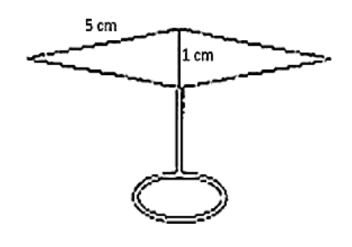# Find the area of the blades of the magnetic compass shown in figure given below:

Question:

Find the area of the blades of the magnetic compass shown in figure given below:Solution:

Area of the blades of magnetic compass = Area of triangle ADB + Area of triangle CDB

Now, for the area of triangle ADB

Perimeter = 2s = AD + DB + BA

2s = 5 cm + 1 cm + 5 cm

s = 5.5 cm

By using Heron's Formula,

Area of the triangle DEF $=\sqrt{s \times(s-a) \times(s-b) \times(s-c)}$

$=\sqrt{5.5 \times(0.5) \times(4.5) \times(0.5)}$

$=2.49 \mathrm{~cm}^{2}$

Also, area of triangle ADB = Area of triangle CDB

Therefore area of the blades of the magnetic compass = 2 × area of triangle ADB

Area of the blades of the magnetic compass = 2 × 2.49

Area of the blades of the magnetic compass $=4.98 \mathrm{~cm}^{2}$